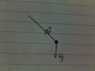# Ball rotated on a string vector diagram

• Ab17

## Homework Statement

is 1222.5i 1 20.2j2 m/s . For that instant, (a) sketch a vector diagram showing the components of its acceler- ation, (b) determine the magnitude of its radial accel- eration, and (c) determine the speed and velocity of the ball.
42. A ball swings counterclockwise in a vertical circle at
the end of a rope 1.50 m long. When the ball is 36.9°
past the lowest point on its way up, its total acceleration is is -22.5i +20.2j m/s2 . For that instant, (a) sketch a vector diagram showing the components of its acceler- ation, (b) determine the magnitude of its radial accel- eration, and (c) determine the speed and velocity of the ball.

a= acì + atj

## The Attempt at a Solution

If the total acceleration is given by -22
5ì + 20.2j m/s2 then isn't 22.5 is the radial acceleration and 20.2m/s2 is the tangential acceleration. I am confused can you please show me the vector diagram too

Hi Ab,

Where did you find that relevant equation ?

Why don't you start drawing a picture of the situation. And work it around to a free body diagram of the ball.

The equation is in vector form

There is tension and gravity

The equation is in vector form
In the sense that it gives an x component and a y component.
There is tension and gravity

• FBD

#### Attachments

•23052016895.jpg
24.7 KB · Views: 437
That's a start. There is a bit more information: 36.9##^\circ##, 1.5 m and ##\vec a = -22.5 \,\hat\imath + 20.2\, \hat\jmath ## m/s2. (In fact I find these values a bit strange: I would expect a negative vertical component. Brings me to the question: what are ##\hat\imath## and ## \hat\jmath## ? I don't see them in the drawing).

Now you need a relevant equation to link the forces and the acceleration. Any idea ?

Last edited:
F= ma
The tension is causing the centripetal acc

So the centripetal acc will be in direction of tension and towards the center

I and j are unit vectors

I and j are unit vectors
Yes, that's why they have a hat. But so far you didn't reveal which way they point.
So the centripetal acc will be in direction of tension and towards the center
Correct. It is a given that the ball describes a circular trajectory. Not with a constant speed (why not?).
Now it's time for another equation. And perhaps you can already draw the figure that part (a) asks for ?

I find these values a bit strange: I would expect a negative vertical component
This was a bit premature. Please ignore.

A ball swings counterclockwise in a vertical circle at
the end of a rope 1.50 m long. When the ball is 36.9° past the lowest point on its way up
I'm not good at approval stamping. But I am good at asking nasty questions, so:
• What makes you think the ##\hat \imath## and ##\hat \jmath## are radial and tangential and not simply horizontal and vertical ?
• Where is that 36.9° angle sitting ?

Because If a is the total acceleration then its components have to be I and J

36.9 is below the x axis

Because If a is the total acceleration then its components have to be I and J
Agree. But this goes for horizontal and vertical just as well.
36.9 is below the x axis
It cleary says something else altogether.

It tells us the location of the ball isnt

When the ball is 36.9° past the lowest point on its way up
to me means the angle between the negative vertical axis (the ##\ -\bf \hat\jmath\ ## direction) is 36.9° .
So the complement of the angle you draw.

So i should break centripetal acc into components or somthn like that

Sort of. You know the ball describes a circular trajectory. There is an equation needed to link acceleration and speed. At the moment we don't have any relevant equations at all. I hope by now ee do agree that only two forces work on the ball. And we know the resultant acceleration. To link ##\vec T + m\vec g## to the given resultant acceleration we also need a relevant equation.
Set up a plan of approach and carry it out !

Break mg into components and then t-mgcos@ = mv2/r and the other component is responsible for tangential accleration so mgsin@ = ma
gsin@ = a

Good plan? Does it work and give you the desired answers ? I doubt it.

However, the ##|\vec F_{\rm centripetal} | = {mv^2\over r}\ ## is an essential equation indeed!

I see two different unknown a with the same symbol. Recipe for disaster.

And I don't see the known ##\ \vec a = -22.5 \hat\imath + 20.2 \hat\jmath\ ## in your plan ?

Fortunately you didn't start carrying out the plan yet.

For your next post I hope to see a plan + execution(or at least attempt at ..)

But: we're getting under way !

ac = v2/r

Mgcos@ = Mac
gcos@ = ac
ac = 7.84m.s2

ac = v2/r
V = sqrt(ac.r)
V = 3.43 m-s1

ac = v2/r
You mean ac = v2/r
##g\cos\alpha= a_c##
##a_c = ## 7.84 m.s2
So T is not contributing and we can forget the given ##
\ \vec a = -22.5 \hat\imath + 20.2 \hat\jmath\
## ?
Furthermore it is m/s2, not m.s2
For your next post I hope to see a plan + execution(or at least attempt at ..)
Perhaps try to keep them separate.